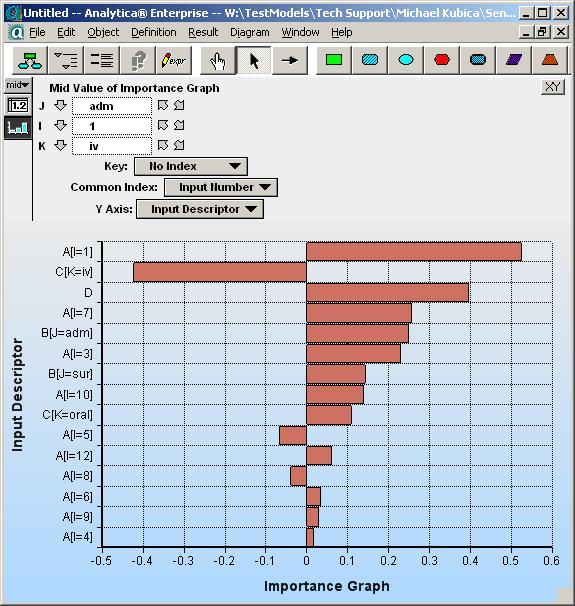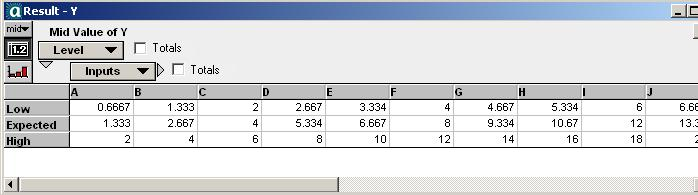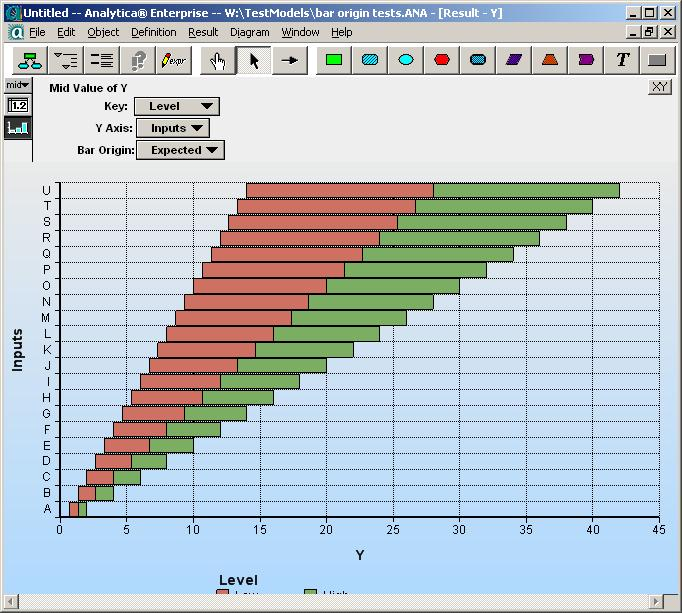(Up to Analytica Graph Settings or Bar Origin)

A "Tornado Plot" is a kind of bar-chart showing the sensitivities or deviations of result due to a list of uncertain inputs. The bars are horizontal, each labeled by the corresponding input variable, and sorted with the largest bars at the top down to the shortest at the bottom -- giving the impression of a tornado funnel cloud.

In @Risk, Tornado plots display the step-wise regression coefficient between and input and the selected output. Only one bar exists for each input variable, and each bar starts at the zero-origin. For this style Tornado, the variable Bar Origin option is unnecessary.

Steps required are:

1. Compute the step-wise regression coefficients.
2. Sort by absolute value (largest first)
3. Truncate to the number of bars desired.
4. Plot result as bar chart, with Swap XY on, and Y-axis in reverse order.

The more common style of Tornado plots displays two bar segments for each input, "Low" and "High", each emanating from the baseline value (which is necessarily between Low and High). There are at least three ways to plot this style of tornado, where the appropriate technique depends on the form of your computed data.

The following is an example of a tornado produced in this fashion. Notice the absence of a bar-origin.## Technique 1

Let:

Index Level := ["Low", "Baseline", "High"]
Index Inputs := ... { a list of inputs }
Variable Y := ... {result indexed by Inputs, Levels, and perhaps others }Also, it is assumed that Inputs has been sorted with the largest variation first, and truncated if desired to only those inputs that one desires to show.

Steps:

1. Plot Y, pivot Inputs to X-axis, Level to Key.
2. Set Chart Style to Bar
3. Select "Variable origin"
Swap XY is set and Bar Overlap changed to 100% automatically. Leave those.
4. On Axis settings, reverse order for Y-axis (result).
5. In graph view, bar origin pulldown appears. Select "Baseline" for the origin.## Technique 2

Let:

Index Level := ["Low", "High"]
Inputs := (a list of inputs)
Variable Baseline := ... { the baseline value, not indexed by Level }
Variable Tornado := ... { sensitivity result, indexed by Level }

Here Tornado might be computed using WhatIfAll, for example.

The goal here is to display a Tornado plot when the Tornado variable's result graph is viewed.

Steps:

1. Show result for Tornado. Ensure you are in edit mode.
2. Click XY button at top right and click "Use Another Variable or Expression".
3. Click Add... and select Baseline. OK
4. In Graph Setup, select Bars, Variable Origin (and Swap XY, and Overlap = 100%) Apply
5. In result graph view, pivot Y-axis = inputs, Key = Level, Bar Origin = Baseline.

Here the origin is the baseline, and the "tops" of the bars are the High/Low values.

## Technique 3

Same model as Technique 2, but here the goal is to display the Tornado when the result for Baseline is viewed.

Steps:

1. Show result for Baseline. Ensure you are in edit mode.
2. Click XY button and click "Use Another Variable or Expression".